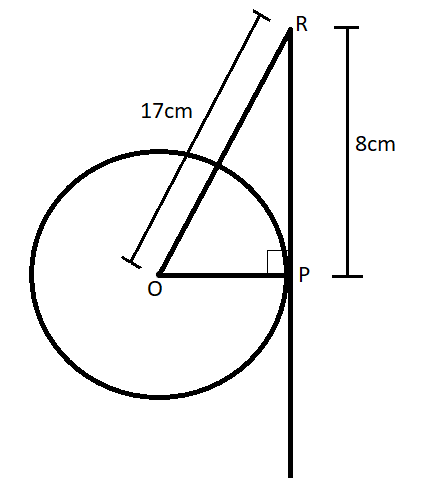QuestionAnswers

# The length of the tangent to a circle from a point 17cm from its center is 8cm. Find the radius of the circle.

Hint: We know that tangent is always perpendicular to the radius made from that point, using this point we’ll use the property of right-angled triangle so formed.
After using the property we’ll get an equation and solving that equation we’ll get the value of the radius of the circle.

Given data: $Length{\text{ }}of{\text{ }}tangent = 8cm$
The distance of the point from centre=17cm
Let the centre of the circle be ‘O’ and the point from which the tangent is made be ‘R’, the point tangent touches the circle be ‘P’.We know that tangents are always perpendicular to the radius made from that point.
Therefore, $OP \bot PR$ or $\angle P = {90^ \circ }$
Using Pythagoras theorem
i.e., in a right-angled triangle ABC with right angle at A, $B{C^2} = A{B^2} + C{A^2}$
Therefore, Using Pythagoras theorem in triangle OPR where $\angle P = {90^ \circ }$
$\Rightarrow O{R^2} = P{R^2} + P{O^2}$
Substituting the value of OR and PR
$\Rightarrow {17^2} = {8^2} + P{O^2}$
On Separating the unknown term,
$\Rightarrow P{O^2} = {17^2} - {8^2}$
On squaring we get,
$\Rightarrow P{O^2} = 289 - 64$
$\Rightarrow P{O^2} = 225$
Taking positive square root on both sides, we get,
$\therefore PO = 15cm$
Therefore, the radius of the circle is 15 cm.

Note: Here we have given the tangent of the circle, so let us discuss some properties related to the tangent of a circle
1.A tangent of a circle always touches the circle at a single point.
2.Tangent is always perpendicular to the radius made at the point of tangency.
3.The length of two tangents drawn to a single point to a circle is always equal.